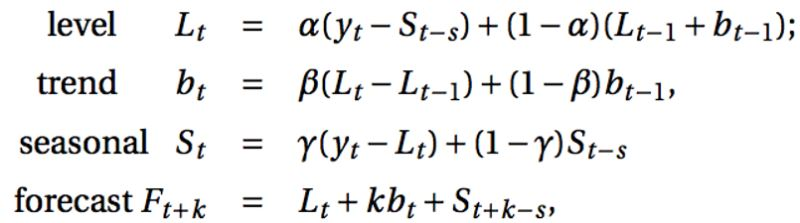## 时间序列之holt-winters（三次指数平滑）

2020/7/24 11:21:05 文章标签: 测试文章如有侵权请发送至邮箱809451989@qq.com投诉后文章立即删除``````# import needed packages
#-----------------------

import math
import numpy  as np
import pandas as pd

from sklearn        import linear_model
from scipy.optimize import fmin_l_bfgs_b

# bring in the passenger data from HW4 to test the function against R output
#---------------------------------------------------------------------------
tsA=[12.7, 14.2, 9.6, 9.2, 10.3, 9.3, 7.8, 7.7, 7.4, 6.9, 6.7, 6.9, 6.6]
# define main function [holtWinters] to generate retrospective smoothing/predictions
#-----------------------------------------------------------------------------------

def holtWinters(ts, p, sp, ahead, mtype, alpha = None, beta = None, gamma = None):
'''HoltWinters retrospective smoothing & future period prediction algorithm
both the additive and multiplicative methods are implemented and the (alpha, beta, gamma)
parameters have to be either all user chosen or all optimized via one-step-ahead prediction MSD
initial (a, b, s) parameter values are calculated with a fixed-period seasonal decomposition and a
simple linear regression to estimate the initial level (B0) and initial trend (B1) values
@params:
- ts[list]:      time series of data to model
- p[int]:        period of the time series (for the calculation of seasonal effects)
- sp[int]:       number of starting periods to use when calculating initial parameter values
- ahead[int]:    number of future periods for which predictions will be generated
- mtype[string]: which method to use for smoothing/forecasts ['additive'/'multiplicative']
- alpha[float]:  user-specified level  forgetting factor (one-step MSD optimized if None)
- beta[float]:   user-specified slope  forgetting factor (one-step MSD optimized if None)
- gamma[float]:  user-specified season forgetting factor (one-step MSD optimized if None)
@return:
- alpha[float]:    chosen/optimal level  forgetting factor used in calculations
- beta[float]:     chosen/optimal trend  forgetting factor used in calculations
- gamma[float]:    chosen/optimal season forgetting factor used in calculations
- MSD[float]:      chosen/optimal Mean Square Deviation with respect to one-step-ahead predictions
- params[tuple]:   final (a, b, s) parameter values used for the prediction of future observations
- smoothed[list]:  smoothed values (level + trend + seasonal) for the original time series
- predicted[list]: predicted values for the next @ahead periods of the time series
sample calls:
results = holtWinters(ts, 12, 4, 24, 'additive')
results = holtWinters(ts, 12, 4, 24, 'multiplicative', alpha = 0.1, beta = 0.2, gamma = 0.3)'''

a, b, s = _initValues(mtype, ts, p, sp)

if alpha == None or beta == None or gamma == None:
ituning   = [0.1, 0.1, 0.1]
ibounds   = [(0,1), (0,1), (0,1)]
optimized = fmin_l_bfgs_b(_MSD, ituning, args = (mtype, ts, p, a, b, s[:]), bounds = ibounds, approx_grad = True)
alpha, beta, gamma = optimized

MSD, params, smoothed = _expSmooth(mtype, ts, p, a, b, s[:], alpha, beta, gamma)
predicted = _predictValues(mtype, p, ahead, params)

return {'alpha': alpha, 'beta': beta, 'gamma': gamma, 'MSD': MSD, 'params': params, 'smoothed': smoothed, 'predicted': predicted}

def _initValues(mtype, ts, p, sp):
'''subroutine to calculate initial parameter values (a, b, s) based on a fixed number of starting periods'''

initSeries = pd.Series(ts[:p*sp])

# print(p)
rawSeason  = initSeries - initSeries.rolling(window = p, min_periods = p, center = True).mean()
initSeason = [np.nanmean(rawSeason[i::p]) for i in range(p)]
initSeason = pd.Series(initSeason) - np.mean(initSeason)
deSeasoned = [initSeries[v] - initSeason[v % p] for v in range(len(initSeries))]
else:
rawSeason  = initSeries / initSeries.rolling(window = p, min_periods = p, center = True).mean()
initSeason = [np.nanmean(rawSeason[i::p]) for i in range(p)]
initSeason = pd.Series(initSeason) / math.pow(np.prod(np.array(initSeason)), 1/p)
deSeasoned = [initSeries[v] / initSeason[v % p] for v in range(len(initSeries))]

lm = linear_model.LinearRegression()
lm.fit(pd.DataFrame({'time': [t+1 for t in range(len(initSeries))]}), pd.Series(deSeasoned))
return float(lm.intercept_), float(lm.coef_), list(initSeason)

def _MSD(tuning, *args):
'''subroutine to pass to BFGS optimization to determine the optimal (alpha, beta, gamma) values'''

predicted = []
mtype     = args
ts, p     = args[1:3]
Lt1, Tt1  = args[3:5]
St1       = args[:]
alpha, beta, gamma = tuning[:]
for t in range(len(ts)):

Lt = alpha * (ts[t] - St1[t % p]) + (1 - alpha) * (Lt1 + Tt1)
Tt = beta  * (Lt - Lt1)           + (1 - beta)  * (Tt1)
St = gamma * (ts[t] - Lt)         + (1 - gamma) * (St1[t % p])
predicted.append(Lt1 + Tt1 + St1[t % p])
else:
Lt = alpha * (ts[t] / St1[t % p]) + (1 - alpha) * (Lt1 + Tt1)
Tt = beta  * (Lt - Lt1)           + (1 - beta)  * (Tt1)
St = gamma * (ts[t] / Lt)         + (1 - gamma) * (St1[t % p])
predicted.append((Lt1 + Tt1) * St1[t % p])

Lt1, Tt1, St1[t % p] = Lt, Tt, St

return sum([(ts[t] - predicted[t])**2 for t in range(len(predicted))])/len(predicted)

def _expSmooth(mtype, ts, p, a, b, s, alpha, beta, gamma):
'''subroutine to calculate the retrospective smoothed values and final parameter values for prediction'''

smoothed = []
Lt1, Tt1, St1 = a, b, s[:]

for t in range(len(ts)):

Lt = alpha * (ts[t] - St1[t % p]) + (1 - alpha) * (Lt1 + Tt1)
Tt = beta  * (Lt - Lt1)           + (1 - beta)  * (Tt1)
St = gamma * (ts[t] - Lt)         + (1 - gamma) * (St1[t % p])
smoothed.append(Lt1 + Tt1 + St1[t % p])
else:
Lt = alpha * (ts[t] / St1[t % p]) + (1 - alpha) * (Lt1 + Tt1)
Tt = beta  * (Lt - Lt1)           + (1 - beta)  * (Tt1)
St = gamma * (ts[t] / Lt)         + (1 - gamma) * (St1[t % p])
smoothed.append((Lt1 + Tt1) * St1[t % p])

Lt1, Tt1, St1[t % p] = Lt, Tt, St

MSD = sum([(ts[t] - smoothed[t])**2 for t in range(len(smoothed))])/len(smoothed)
return MSD, (Lt1, Tt1, St1), smoothed

'''subroutine to generate predicted values @ahead periods into the future'''

Lt, Tt, St = params
return [Lt + (t+1)*Tt + St[t % p] for t in range(ahead)]
else:
return [(Lt + (t+1)*Tt) * St[t % p] for t in range(ahead)]

# print out the results to check against R output
#------------------------------------------------

results = holtWinters(tsA, 4, 4, 5, mtype = 'additive')
# # results = holtWinters(tsA, 12, 4, 24, mtype = 'multiplicative')

print("TUNING: ", results['alpha'], results['beta'], results['gamma'], results['MSD'])
# # print('----')
print("FINAL PARAMETERS: ", results['params'])
print("PREDICTED VALUES: ", results['predicted'])
``````

暂无相关的数据...